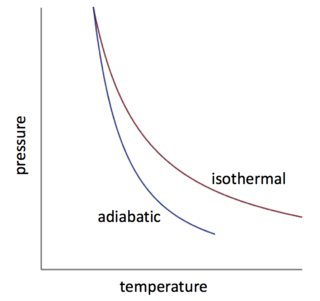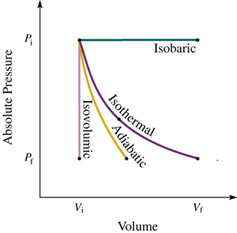# IMAT 2017 Q59 [Ideal Gas Change]

A fixed mass of an ideal gas undergoes a change in which it is supplied with 3500 J of thermal energy. At the same time this gas does 3500 J of work on its surroundings.

Which type of change does the gas undergo during this time?

B. isothermal
C. isochoric
D. isomeric
E. isobaric

2 Likes

The basic processes with ideal gas are known as follows: isothermal, isochoric, isobaric, and adiabatic.

In these systems, one or more variables is held constant.

1. Isobaric; where the pressure of the system is kept constant. \delta P=0

1. Isochoric; where the volume of the system is kept constant. \delta V=0

1. Isothermal; where the temperature of the system is kept constant. \delta U=0 and \delta T=0

Note that when \delta T=0, the relation between Volume and Pressure is inversely proportional.

1. Adiabatic; where no heat is being added or removed from the system (no heat transfer). Q= 0A guide to all 4 processes: